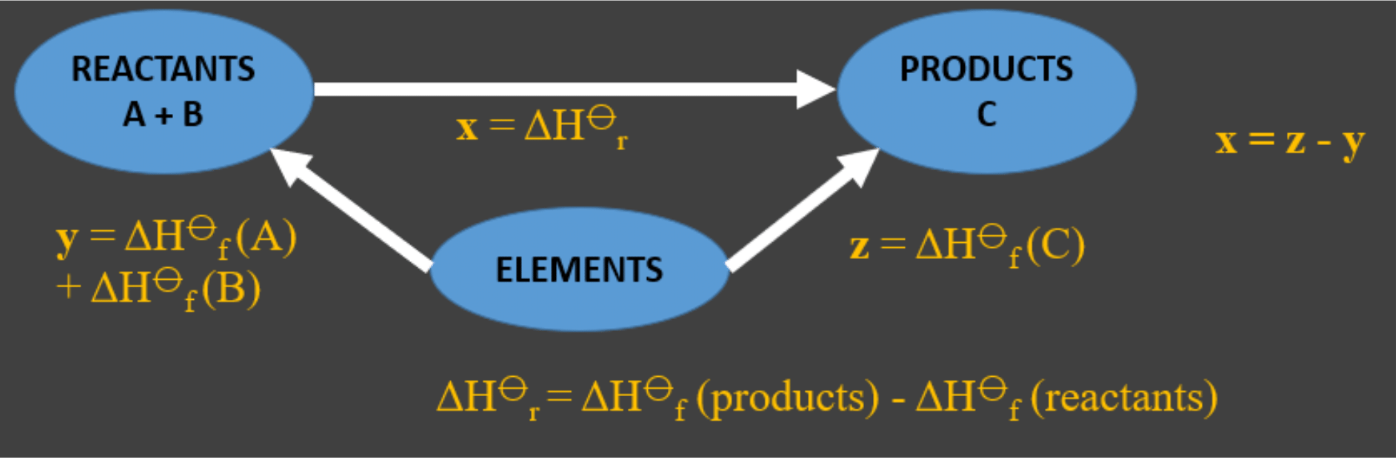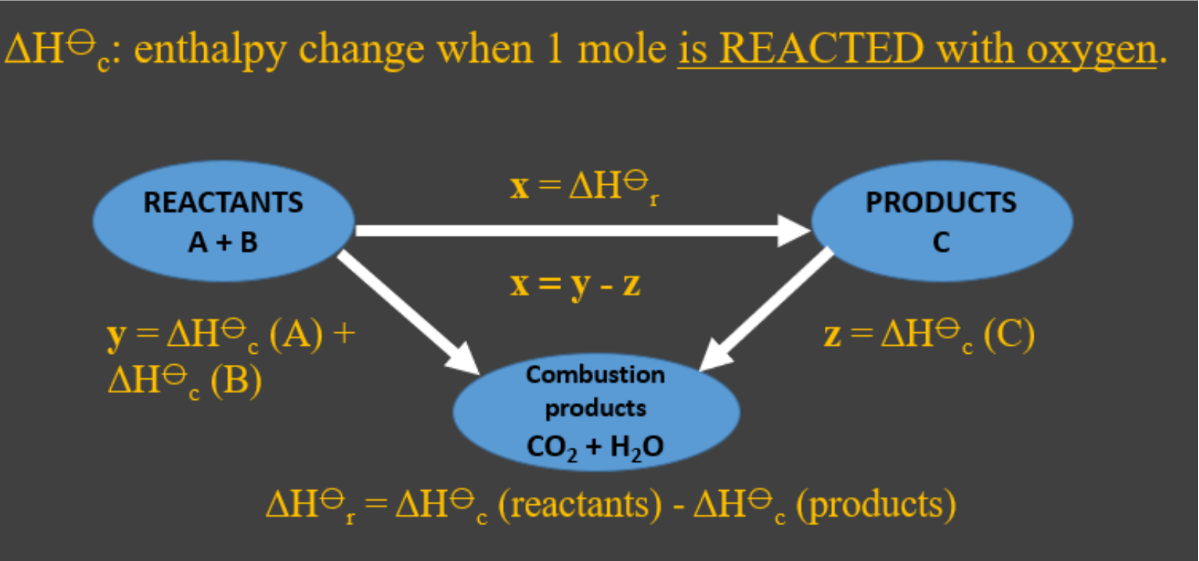# Enthalpy: Hess's Law#### All You Need in One Place

Everything you need for better marks in primary, GCSE, and A-level classes.#### Learn with Confidence

We’ve mastered the UK’s national curriculum so you can study with confidence.#### Instant and Unlimited Help

0/4
##### Intros
###### Lessons
1. Measuring enthalpy change: Hess's law
2. Hess's law.
3. Using Hess's law to build enthalpy cycles.
4. WORKED EXAMPLE: Find the enthalpy change using enthalpy of formation.
5. WORKED EXAMPLE: Find the enthalpy change using enthalpy of combustion.
0/0
##### Examples
###### Topic Notes

In this lesson, we will learn:

• To recall Hess's law and understand how it relates to enthalpy change of chemical reactions.
• To use Hesss law to construct enthalpy cycles.
• To use enthalpy cycles and the standard enthalpy of formation to calculate the enthalpy change of chemical reactions.

Notes:

• There are several ways to find the enthalpy change of a reaction. In the next few lessons we will look at ways to find the enthalpy change based on what happens to heat energy in reactions and what it does as a result.

• Hess's law states that the enthalpy change of a reaction is independent of the route taken (to get from reactants to products).
This solves a lot of problems for chemists because many enthalpy changes cant be measured directly!

• Using Hess's law, we can find the overall enthalpy change of a reaction in the same way that a car gets from A $\,$$\,$ B by taking a detour from A $\,$$\,$ C and then C $\,$$\,$ B, instead of the direct route.
• In a car journey, whether you made two, five or zero stops along the way, the overall change in your position from where you start and where you finish is still the same. In physics, this overall change in position is called the displacement.
• In chemistry, if we cant do a given chemical reaction and go directly from reactants to products, we can take a chemical detour to get there. If we start and finish at the same substances, the overall change in enthalpy will still be the same it doesnt matter which route we take.

We can create a Hess cycle using the reactants, products and their constituent elements using the standard enthalpy of formation.
In Introduction to enthalpy, we learned the definition of standard enthalpy of formation: The enthalpy changes when one mole of a substance is formed from its constituent elements in their standard states.
This is the enthalpy change associated with going from constituent elements to chemical compounds. This is the detour we can use!By using the constituent elements as the detour, we can still find the enthalpy change of reaction using the standard enthalpy of formation of the reactants and products. This cycle constructed obeys Hesss law.

Let x = enthalpy change of the reaction, y = standard enthalpy of formation of reactants A and B, and z = standard enthalpy of formation of product C.
$\triangle H^{\ominus}$r = $\triangle H^{\ominus}$f (products) - $\triangle H^{\ominus}$f (reactants)

The sign of the enthalpy change is vital and must be included in your answer.
• Enthalpy of formation is the heat energy change of the molecules, not the environment.
• This means if the enthalpy change is positive, the reaction is endothermic because the molecules have gained energy overall.
• If the enthalpy change is negative, the reaction is exothermic. because the molecules have lost energy overall.

• You can also use Hess cycles with enthalpy of combustion data, but they have an important difference to enthalpy of formation cycles. The detour arrows are reversed.

•The direction of the arrows in the Hess cycle is very important ‐ its related to the enthalpy definition. Enthalpy of combustion is reacting a substance; formation is forming it. Thats why they are the opposite way around to each other.

• WORKED EXAMPLE: Using Hess cycles to find enthalpy of reaction.

• The reaction of ethene and hydrogen to produce ethane is shown by the following equation:

C2H4 (g) + H2 (g) $\,$$\,$ C2H6 (g)

The enthalpy of formation ($\triangle H^{\ominus}$f) data1 for the three compounds is as follows:
C2H6: -84.68 kJ/mol
H2: 0 kJ/mol (H2 is the standard state elemental form)
C2H4: +52.26 kJ/mol

Use this data to find the enthalpy change, $\triangle H$r, for this reaction
.

Using the $\triangle H^{\ominus}$f data we can construct a Hess cycle, or enthalpy cycle, with the elements as the middle or detour step. See the image below:

The blue arrows in the image show our route from the reactants to products. It has two steps:
• We go from reactants to the elements. We are given $\triangle H^{\ominus}$f for the reactants, but we arent forming the reactants, we are going back to the elements. This means we reverse the sign of the reactant values.
• We go from elements to the products. We have $\triangle H^{\ominus}$f for the products and we are going from the elements to the products, AKA forming the substances. So the sign remains the same.
These steps give us a calculation for the enthalpy change of the reaction:

$\triangle H$r = $\triangle H^{\ominus}$f (products) - $\triangle H^{\ominus}$f (reactants)

Using our values we can find:

$\triangle H$r = -84.68 (0 + 52.26)

$\triangle H$r =-84.68 52.26 = -136.94 kJ/mol

You must include a sign and units with your answer, even a positive sign!

• Hess cycles can also be used in this way for enthalpy of combustion ($\triangle H^{\ominus}$c ) data.
• With enthalpy of combustion data, your detour uses the combustion products H2O (l) and CO2 (g). You can use it to find the $\triangle H^{\ominus}$r in a reaction, or the $\triangle H^{\ominus}$f of a substance.
• Enthalpy of combustion arrows in a Hess cycle will always point downward to the combustion products!
• With Hess cycles using enthalpy of combustion, pay attention to your stoichiometry (the number of moles of each reactant/product). You need to balance this like any other equation and multiply the enthalpy values by the number of moles in the reaction equation.

• WORKED EXAMPLE: Using enthalpy of combustion data and Hess cycles to find enthalpy change.

• The reaction below describes the formation of ethane, C2H6, from its constituent elements:

2C (s) + 3H2 (g) $\,$$\,$ C2H6 (g)

The enthalpy of formation ($\triangle H^{\ominus}$c ) data2 for the substances involved is below:
C: -394 kJ/mol
H2: -286 kJ/mol
C2H6: -1560 kJ/mol

Use this data to construct a Hess cycle and calculate the enthalpy of formation ($\triangle H^{\ominus}$f) of ethane, C2H6
.

Construct a Hess cycle like before, with the reaction equation at the top. This time, the difference is that we use complete combustion products (CO2 and H2O) as the detour step. See the image below, particularly the blue arrows showing the route and the black arrows showing the combustion enthalpy data and the direction they point in.

The important difference between this enthalpy cycle and the previous worked example is the direction of the enthalpy arrows.
• When using the enthalpy of formation data (previous example) the enthalpy arrows point up because we go from elements to the chemical substances. This gives us an overall working out of $\triangle H^{\ominus}$r = $\triangle H^{\ominus}$f (products) - $\triangle H^{\ominus}$f (reactants).
• In this example, we use enthalpy of combustion, where the arrows will always point downward to the combustion products!
Using the blue arrows in this example, we can show a route from reactants to products and it works out with the following calculation:

$\triangle H^{\ominus}$r = $\triangle H^{\ominus}$c (reactants) - $\triangle H^{\ominus}$f (products)

For our example, we are making one mole of ethane from its constituent elements so we can change the $\triangle H$r symbol for $\triangle H^{\ominus}$f. Using the data we have then, we can make the calculation:

$\triangle H^{\ominus}$f (C2H6) = ((2*-394) + (3*-286)) - (-1560) = -86 kJ / mol

1 Source for standard enthalpy of formation data: ATKINS, P. W., & DE PAULA, J. (2006).Atkins' Physical chemistry. Oxford, Oxford University Press.

2 Source for enthalpy of combustion and enthalpy of formation data: https://www.york.ac.uk/media/chemistry/teachinglabs/instruments/instructions/DATABOOK%20FINAL%20VERSION%20for%20web%202011-12.pdf